0
9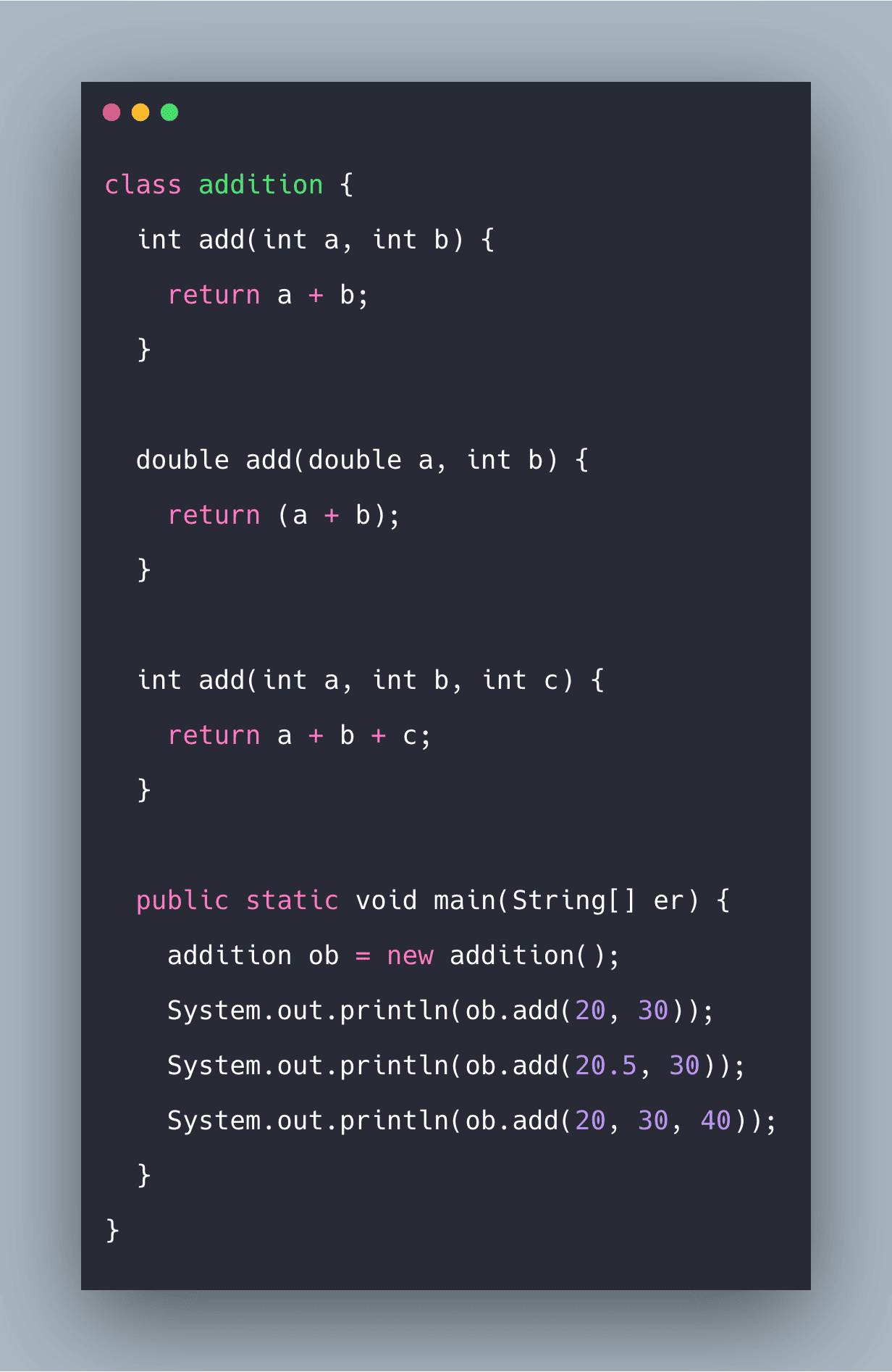Method overloading in Java means a class is having multiple methods with the same name but different in the parameter. Suppose you want to write an extensive program which contains many methods, remembering every method name might be difficult. So you can name every method with the same name but using different parameters. This is the coolness of method overloading.

1. The name of all the methods should be the same but data type should be different from the parameters.
2. If you took (int, int) as the data type for the parameters than for calling that method the data type should be (int, int) like (20,30).
3. If you are using int method_name than there should be a return statement inside that method.Whereas in the case of void method_name, there is no need for the return statement.
4. If you are using double as a parameter inside a method, then the data type for the method should be double. This means it should be equal to or higher than the data type used in the parameters. See the following example code.
`double add(int a, int b, double c)`

Method Overloading is the feature that allows the class to have more than one method having the same name if their argument lists are different. It is similar to a constructor overloading in Java, that allows the class to have more than one constructor having different argument lists.

```class A
{
{

}
{

}
}
```

See the following program.

```class addition {
int add(int a, int b) {
return a + b;
}

double add(double a, int b) {
return (a + b);
}

int add(int a, int b, int c) {
return a + b + c;
}

public static void main(String[] er) {
}
}```

See the output.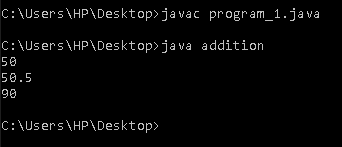### Explanation

In the above program, the method name is the same for all three methods, i.e., add( ), but their parameters are different in every method. Like in first method add(int , int) , in second add(double , int) and in third add(int, int ,int). Remember that if you are taking double as a parameter than the method data type should be double too.

## #Different ways to overload the method in Java

1. By changing the number of arguments in the method.
2. By changing the data type of the method.
3. By changing the order of the parameter.

### By changing several arguments/parameters in the method

A different method can be overloaded by changing the number of arguments in the method.

See the following program.

```class new_add {
int add(int a, int b) /* 2 arguments */
{
return a + b;
}

int add(int a, int b, int c) /* 3 arguments */
{
return a + b + c;
}

public static void main(String[] er) {
}
}```

See the output.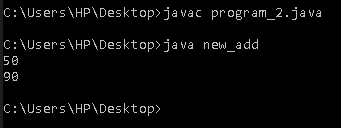### #Explanation

In the above program, the first method has two arguments, i.e. (int a, int b) whereas in the second method, three arguments, i.e. (int a, int b, int c). Therefore we can overload the method using different arguments or parameters.

### #By changing the data type of the arguments

Suppose there are two methods with the same name then we can distinguish them with the help of different data types present in that method.

See the following program.

```class data_add {
int add(int a, int b) /* 2 arguments */
{
return a + b;
}

double add(double a, int b) /* 2 arguments */
{
return a + b;
}

public static void main(String[] er) {
}
}```

See the output.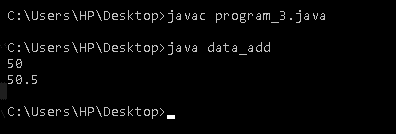### #Explanation

In the above program, both the methods are having two arguments, but they have a different data type. That is one is (int, int ) and another is (double, int).

### #By changing the order of the parameter

See the following program.

```class order_add {
double add(int a, double b) /* 2 arguments */
{
return a + b;
}

double add(double a, int b) /* 2 arguments */
{
return a + b;
}

public static void main(String[] er) {
}
}```

See the output.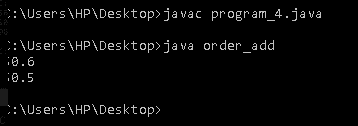### #Explanation

For the first method, the order is (int, double ) whereas, for the second method, the order is(double, int). So you can do method overloading by changing the order of the argument also.

The benefit of the static keyword in method overloading is that there is no need for creating an object inside the main method. You can directly call the method using class_name.method_name.

See the following program.

```class sum {
static int add(int a, int b) {
return a + b;
}

static double add(double a, int b) {
return (a + b);
}

static int add(int a, int b, int c) {
return a + b + c;
}

public static void main(String[] er) {
}
}```

See the output.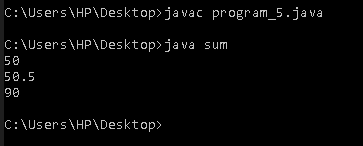We can overload the main method like a straightforward method.

See the following program.

```class main_overload {
public static void main() {
}

public static void main(String args[]) {
ob.main();
}
}```

See the output.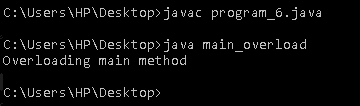Case 1:

```int mymethod(int a, int b, float c)
int mymethod(int var1, int var2, float var3)```

Result: Compile time error. Argument lists are the same. Both methods are having the same number, data types, and the same sequence of data types.

Case 2:

```int mymethod(int a, int b)
int mymethod(float var1, float var2)```

Result: Perfectly fine. Valid case of overloading. Here data types of arguments are different.

Case 3:

```int mymethod(int a, int b)
int mymethod(int num)```

Result: Perfectly fine. Valid case of overloading. Here the number of arguments is different.

Case 4:

```float mymethod(int a, float b)
float mymethod(float var1, int var2)```

Result: Perfectly fine. Valid case of overloading. The sequence of the data types of parameters are different, the first method is having (int, float) and second is having (float, int).

Case 5:

```int mymethod(int a, int b)
float mymethod(int var1, int var2)```

Result: Compile time error. Argument lists are the same. Even though the return type of methods is different, it is not a valid case. Since the return type of a method doesn’t matter while overloading the method.